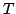Next: Recovery Up: Low-dimensional parameter mapping using Previous: Criteria

Setup of timbre space

Our working measure of timbre will be the one assumed by the bonk~ object available in Pd and Max [PuckettePuckette1998]. The incoming sound is split into 11 frequency bands, three with center frequency 100, 300, and 500 Hz. and bandwidth 200 Hz, and eight more tuned to each half-octave above 500 Hz., so that the top one is centered at 8 kHz. In each band we estimate a loudness contribution as the fourth root of the power on the band; this is close to a loudness measure suggested in [Rossing, Moore, and WheelerRossing et al.2002].

It turns out, of course, that the measured power in these eleven bands is strongly intercorrelated. We decorrelate them in two steps. If the raw timbre vector iswe first rotate the timbre vector into one component equal to(suitably normalized) and ten other orthogonal components to form another timbre-without-loudness vector,, of ten dimensions. We then apply multidimensional scaling toto give yet another timbre vector, in ten dimensions, so that each component ofhas sample mean zero and variance one, and so that the components ofare uncorrelated. To find this transformation, a representative corpus of sounds is analyzed. When analysing synthetic timbres, a systematic sampling is made as each of the usable parameters attain all the values in their domain; for input sounds the corpus is assembled intuitively.

The musician's controlling signal and a database of possible synthetic sounds are both thus analyzed; each of the two requires its own decorrelating transformation. Associated with each synthetic sound, we also store the synthesis parameters that led to the sound so that we can re-create it later.

By normalizing the timbre vectors of both the input and the available outputs to have the same means and variances, we maximize the closeness of fit between the two; this maximizes the likelihood of finding `good' output parameters. In doing this we are dropping any promise of making the output timbre imitate the input timbre exactly; they should move in roughly the same directions, but each according to its natural span.Next: Recovery Up: Low-dimensional parameter mapping using Previous: Criteria
Miller Puckette 2005-05-28